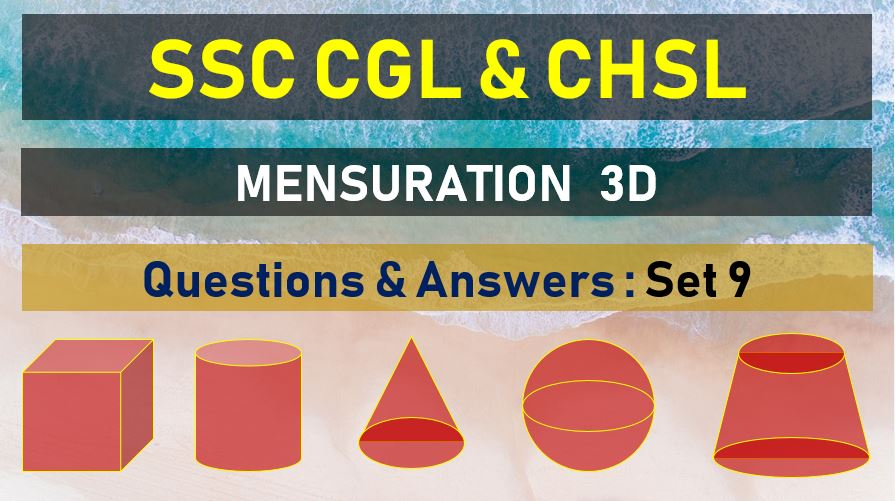Tuesday, June 22, 2021
Home > Latest Announcement > SSC CGL Surface Area and Volume Questions Answers Set 9

# SSC CGL Surface Area and Volume Questions Answers Set 9SSC Mensurations 3D
Surface Area & Volume Questions Sets
Set 1Set 2Set 3Set 4Set 5
Set 6Set 7Set 8Set 9Set 10

SSC CGL Surface Area and Volume Questions Answers Set 9: Ques No 1

If the height and the radius of the base of a cone are each increased by 100%, then the volume of the cone become

Options:

A. double that of the original

B. three times that of the original

C. six times that of the original

D. eight times that of the original

Answer: D

Solution:

SSC CGL Surface Area and Volume Questions Answers Set 9: Ques No 2

If the height of a right circular cone is increased by 200% and the radius of the base is reduced by 50%, the volume of the cone

Options:

A. increases by 25%

B. increases by 50%

C. remains unaltered

D. decreases by 25%

Answer: D

Solution:

SSC CGL Surface Area and Volume Questions Answers Set 9: Ques No 3

A cone of height 15 cm and base diameter 30 cm is carved out of a wooden sphere of radius 15 cm. The percentage of used wood is :

Options:

A. 75%

B. 50%

C. 40%

D. 25%

Answer: D

Solution:

SSC CGL Surface Area and Volume Questions Answers Set 9: Ques No 4

If both the radius and height of a right circular cone are increased by 20%, its volume will be increased by

Options:

A. 20%

B. 40%

C. 60%

D. 72.8%

Answer: D

Solution:

SSC CGL Surface Area and Volume Questions Answers Set 9: Ques No 5

Each of the height and base radius of a cone is increased by 100%. The percentage increase in the volume of the cone is

Options:

A. 700%

B. 400%

C. 300%

D. 100%

Answer: A

Solution:

SSC CGL Surface Area and Volume Questions Answer Set 9: Ques No 6

If radius of a cylinder is decreased by 50% and the height is increased by 50% to from a new cylinder, the volume will be decreased by

Options:

A. 6%

B. 25%

C. 62.5%

D. 75%

Answer: C

Solution:

SSC CGL Surface Area and Volume Questions Answer Set 9: Ques No 7

A cone of height 7 cm and base radius 1 cm is carved from a cuboidal block of wood 10 cm x 5 cm x 2 cm

Options:

A. 278/3%

B. 139/3%

C. 127/2%

D. 124/3%

Answer: A

Solution:

SSC CGL Surface Area and Volume Questions Answer Set 9: Ques No 8

On increasing each side of a squire by 50%, the ratio of the area of new formed square and the given square will be

Options:

A. 9 : 5

B. 9 : 7

C. 9 : 3

D. 9 : 4

Answer: D

Solution:

SSC CGL Surface Area and Volume Questions Answer Set 9: Ques No 9

A plane divided a right circular cone into two parts of equal volume . if the plane is parallel to the base, then the ratio in which the height of the cone is divided, is

Options:

A. 1:√2

B. 1:3√2

C. 1:3√2-1

D. 1:3√2+1

Answer: C

Solution:

SSC CGL Surface Area and Volume Questions Answer Set 9: Ques No 10

A large solid sphere is melted and molded to form identical right circular cones with base radius and height same as the radius of the sphere. One of these cones is melted and molded to form a smaller solid sphere. Then the ratio of the surface area of the smaller to the surface area of the larger sphere is

Options:

A. 1: 34/3

B. 1: 23/2

C. 1:32/3

D. 1:33/4

Answer: D

Solution:

SSC Mensurations 3D
Surface Area & Volume Questions Sets
Set 1Set 2Set 3Set 4Set 5
Set 6Set 7Set 8Set 9Set 10
error: Content is protected !!